Next: 4.2.2.1 Boundary Flux Equation Up: 4.2 Supported Model Structures Previous: 4.2.1 Volume Models

## 4.2.2 Boundary Models

Analogously to the situation for the volume model equations, a generalized formulation of models describing the flux of quantities across the interface between two adjacent segments has been discretized, leaving the user the possibility to provide models determining the coefficients of the underlying equation system. In the following equations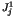denotes the flux of the quantityleaving segment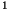whereas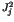gives the flux of quantityentering segment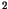(Fig. 4.1).

The default boundary condition (4.6) for all quantities is a homogeneous Neumann type condition which defines a zero flux over the boundary.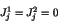(4.6)

These default boundary conditions can be replaced for any combination and number of quantities on both of the adjoining segments by either defining the flux over the interface (Section 4.2.2.1) or defining the actual concentration of the respective quantities by adding Dirichlet type boundary equations as described in Section 4.2.2.2.Next: 4.2.2.1 Boundary Flux Equation Up: 4.2 Supported Model Structures Previous: 4.2.1 Volume Models
Robert Mlekus
1999-11-14